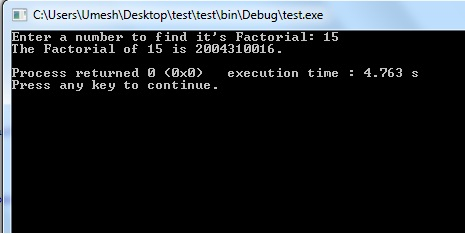Friday , August 23 2019

# Recursion in C Programming

Recursion in C Programming is technique in which function call’s itself number of times. The function calls itself is referred as recursive function and call is recursive call. The recursion continues until some condition is met to prevent it. To prevent infinite recursion control statements or similar approach can be used where one branch makes the recursive call and other doesn’t. Let’s understand the concept of recursion using simplest example.

Example 1: In this example code, recurse() is a recursive function which calls itself. This program executes for forever and prints “This is recursive function”.

Explanation: In this example code, we can see the function recurse(), in its definition calls itself. So, recurse() becomes a recursive function. When we compile example code, the main() function will call recurse(). The recurse() will continue to call itself. The output of this program will continuously print “This is recursive function”. Towards the end of output we’ll get “segmentation fault” or program will be crashed. You might have thought the program would continue executing forever because recursive function recurse() would continue to calling itself forever but it did not happened. This program will crash because of following reasons:

• For each call to recurse(), a new function stack is created.
• Recurse() calling itself continuously, new function stack also are created continuously.
• At one point this causes stack overflow and program gets crashed.

Example 2: Write a program which takes integer number as an argument, and prints factorial of it. Recursion in C Programming is pretty handy technique to solve such problems.

Explanation: Initially factorial() is called from main() function with number passed as an argument. Suppose the value of num is 15 initially. The output is as below:Output of Recursive Factorial Program

Now apart from logic, the question is how recursion works? A recursive function has two parts the base case and recursive call. Base case is the case for which the function is evaluated without recursion. In above example the base case is if(num == 1 || num == 0), which prints value of n without making any subsequent recursive call. Recursive call is the central part of a recursive function and during each call parameter values come closer to the base case. And, when the base case is reached it is evaluated without recursion and results are returned to the previous call, then from previous to previous and so on, until the function reaches to its caller.

Apart from this there are several variant of recursion. These are Linear Recursion, Tail Recursion, and Binary Recursion. These types of recursion tell us making recursive calls in different ways depending upon the problem. We’ll get into them in future lesson. Note, Multiple Recursion can be treated a generalized form of binary recursion. When a function makes multiple recursive calls possibly more than two, it is called multiple recursions.

#### Pros and Cons of Recursion in C Programming

Recursion makes program elegant, cleaner and easier to understand. All algorithms can be defined recursively which makes it easier to visualize and prove. There are some data structures you will see which are quite easy to code with help of recursion. Tree traversals, quick, and merge sort are a few examples. If the speed of the program is vital then, you should avoid using recursion. Recursions use more memory and are generally slow.

This is it for Recursion in C Programming. In this post we haven’t touched on types of recursions but will see their details in future lesson. Considering pros and cons of recursion, you would have realized that recursion is programmer friendly not system because it’s shorter to write, cleaner to see. In next lesson we’ll learn about Pointers in C Programming. Please do write us if you have any suggestion/comment or come across any error on this page. Thanks for reading.

### About Umesh LokhandeUmesh Lokhande holds a Master degree in Scientific Instrumentation from University of Applied Sciences Jena, Germany. and has previously worked at Orbotech, Alere Technologies etc. Umesh is also a founder and first author of BINARYUPDATES.COM